# US20130246496A1 - Floating-point vector normalisation - Google Patents

## Info

Publication number
US20130246496A1
US20130246496A1 US13/825,179 US201113825179A US2013246496A1 US 20130246496 A1 US20130246496 A1 US 20130246496A1 US 201113825179 A US201113825179 A US 201113825179A US 2013246496 A1 US2013246496 A1 US 2013246496A1
Authority
US
United States
Prior art keywords
value
floating point
processing
scaling
components
Prior art date
Legal status (The legal status is an assumption and is not a legal conclusion. Google has not performed a legal analysis and makes no representation as to the accuracy of the status listed.)
Abandoned
Application number
US13/825,179
Inventor
Dominic Hugo Symes
Current Assignee (The listed assignees may be inaccurate. Google has not performed a legal analysis and makes no representation or warranty as to the accuracy of the list.)
ARM Ltd
Original Assignee
ARM Ltd
Priority date (The priority date is an assumption and is not a legal conclusion. Google has not performed a legal analysis and makes no representation as to the accuracy of the date listed.)
Filing date
Publication date
Priority claimed from GB1016071.1 external-priority
Application filed by ARM Ltd filed Critical ARM Ltd
Assigned to ARM LIMITED reassignment ARM LIMITED ASSIGNMENT OF ASSIGNORS INTEREST (SEE DOCUMENT FOR DETAILS). Assignors: NYSTAD, JORN, CRASKE, SIMON, SYMES, DOMINIC HUGO
Publication of US20130246496A1 publication Critical patent/US20130246496A1/en
Abandoned legal-status Critical Current

• 238000010606 normalization Methods 0.000 title abstract description 44
• 230000001276 controlling effect Effects 0.000 claims description 36
• 241000724284 Peanut stunt virus Species 0.000 claims description 34
• 230000000875 corresponding Effects 0.000 claims description 28
• 238000004590 computer program Methods 0.000 claims description 20
• 230000001419 dependent Effects 0.000 claims description 20
• 230000004044 response Effects 0.000 claims description 6
• 238000000034 method Methods 0.000 description 30
• 238000010586 diagram Methods 0.000 description 18
• 230000015654 memory Effects 0.000 description 14
• 238000004364 calculation method Methods 0.000 description 8
• 230000000295 complement Effects 0.000 description 4
• 230000000694 effects Effects 0.000 description 4
• 230000006399 behavior Effects 0.000 description 2
• 238000006243 chemical reaction Methods 0.000 description 2
• 230000003993 interaction Effects 0.000 description 2
• 230000001960 triggered Effects 0.000 description 2

## Images

•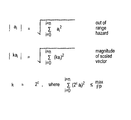•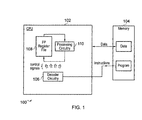•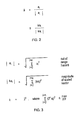•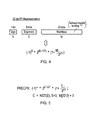•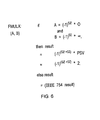•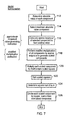•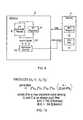•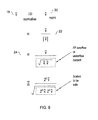•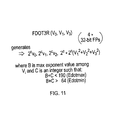•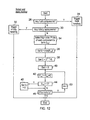•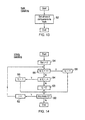•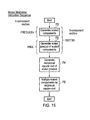•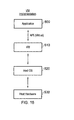## Classifications

• GPHYSICS
• G06COMPUTING; CALCULATING OR COUNTING
• G06FELECTRIC DIGITAL DATA PROCESSING
• G06F17/00Digital computing or data processing equipment or methods, specially adapted for specific functions
• G06F17/10Complex mathematical operations
• GPHYSICS
• G06COMPUTING; CALCULATING OR COUNTING
• G06FELECTRIC DIGITAL DATA PROCESSING
• G06F7/00Methods or arrangements for processing data by operating upon the order or content of the data handled
• G06F7/38Methods or arrangements for performing computations using exclusively denominational number representation, e.g. using binary, ternary, decimal representation
• G06F7/48Methods or arrangements for performing computations using exclusively denominational number representation, e.g. using binary, ternary, decimal representation using non-contact-making devices, e.g. tube, solid state device; using unspecified devices
• G06F7/483Computations with numbers represented by a non-linear combination of denominational numbers, e.g. rational numbers, logarithmic number system or floating-point numbers
• GPHYSICS
• G06COMPUTING; CALCULATING OR COUNTING
• G06FELECTRIC DIGITAL DATA PROCESSING
• G06F7/00Methods or arrangements for processing data by operating upon the order or content of the data handled
• G06F7/38Methods or arrangements for performing computations using exclusively denominational number representation, e.g. using binary, ternary, decimal representation
• G06F7/48Methods or arrangements for performing computations using exclusively denominational number representation, e.g. using binary, ternary, decimal representation using non-contact-making devices, e.g. tube, solid state device; using unspecified devices
• G06F7/544Methods or arrangements for performing computations using exclusively denominational number representation, e.g. using binary, ternary, decimal representation using non-contact-making devices, e.g. tube, solid state device; using unspecified devices for evaluating functions by calculation
• G06F7/552Powers or roots, e.g. Pythagorean sums
• G06F7/5525Roots or inverse roots of single operands
• GPHYSICS
• G06COMPUTING; CALCULATING OR COUNTING
• G06FELECTRIC DIGITAL DATA PROCESSING
• G06F9/00Arrangements for program control, e.g. control units
• G06F9/06Arrangements for program control, e.g. control units using stored programs, i.e. using an internal store of processing equipment to receive or retain programs
• G06F9/30Arrangements for executing machine instructions, e.g. instruction decode
• G06F9/30003Arrangements for executing specific machine instructions
• G06F9/30007Arrangements for executing specific machine instructions to perform operations on data operands
• G06F9/30036Instructions to perform operations on packed data, e.g. vector operations
• GPHYSICS
• G06COMPUTING; CALCULATING OR COUNTING
• G06FELECTRIC DIGITAL DATA PROCESSING
• G06F2207/00Indexing scheme relating to methods or arrangements for processing data by operating upon the order or content of the data handled
• G06F2207/552Indexing scheme relating to groups G06F7/552 - G06F7/5525
• G06F2207/5521Inverse root of a number or a function, e.g. the reciprocal of a Pythagorean sum
• GPHYSICS
• G06COMPUTING; CALCULATING OR COUNTING
• G06FELECTRIC DIGITAL DATA PROCESSING
• G06F5/00Methods or arrangements for data conversion without changing the order or content of the data handled
• G06F5/01Methods or arrangements for data conversion without changing the order or content of the data handled for shifting, e.g. justifying, scaling, normalising
• G06F5/012Methods or arrangements for data conversion without changing the order or content of the data handled for shifting, e.g. justifying, scaling, normalising in floating-point computations

## Abstract

When performing vector normalisation upon floating point values, an approximate reciprocal value generating instruction is used to generate an approximate reciprocal value with a mantissa of one and an exponent given by a bitwise inversion of the exponent field of the input floating point number. A modified number of multiplication instruction is used which performs a multiplication giving the standard IEEE 754 results other than when a signed zero is multiplied by a signed infinity which results a signed predetermined substitute value, such as 2. The normalisation operation may be performed by calculating a scaling value in dependence upon the vector floating point value using the approximate reciprocal value generating instruction. Each of the input components may then be scaled using the modify multiplication instruction to generate a scaled vector floating point value formed of a plurality of scaled components. The magnitude of the scaled vector floating point value can then be calculated and each of the individual scaled components divided by this magnitude to generate a normalised vector floating point value. The scaling value may be set to 2, where C is an integer value selected such that the sum of the squares of the plurality of scale components is less than a predetermined limit value.

## Description

• This invention relates to the field of data processing systems. More particularly, this invention relates to the field of data processing systems supporting vector floating point arithmetic.
• It is known to perform vector normalisation operations upon a floating point vector V to generate a normalised vector that has unit length and points in the same direction as the vector V. This vector normalisation can be performed as the following sequence of calculations:
• 1. Given an input vector V, compute the dot-product of the input vector V with itself;
• 2. Compute the reciprocal square root of the dot-product; and
• 3. Multiply each component of the input vector by the reciprocal square root value.
• While the above sequence of operations work well for idealised mathematical real numbers, there is a problem that floating point numbers only represent mathematical real numbers within a limited range and with a limited degree of precision. A particular problem in the context of the above described vector normalisation technique is that the dot-product may overflow or underflow resulting in at least a loss of precision in the final result and potentially an unacceptable error.
• It is desirable that whatever approach is taken to address this problem, there should not be an additional inaccuracy introduced in the determination of the normalised vector and that the amount of additional overhead, such as circuit area and processing time, should not increase unduly.
• Viewed from one aspect the present invention provides an apparatus for processing data comprising:
• processing circuitry configured to perform processing operations upon data values; and
• decoder circuitry coupled to said processing circuitry and configured to decode program instructions to generate control signals for controlling said processing circuitry to perform processing operations specified by said program instructions; wherein
• said decoder circuitry decodes an approximate reciprocal value generating instruction to generate control signals to control said processing circuitry to perform a processing operation upon a floating point number with an integer exponent value E and a mantissa value M to generate an approximate reciprocal value with an exponent C that is dependent upon E and a mantissa that represents 1.
• The present technique recognises that calculating a reciprocal value of a floating point number is useful when performing normalisation as by multiplying each of the vector components by the reciprocal of the magnitude of the largest of the vector components before the normalisation is performed, the likelihood of overflow or underflow is reduced. However, a problem with this approach is that it may be computationally intensive to compute the reciprocal and there may be a loss of precision associated with the manipulation of the mantissa value of the vector components. The approximate reciprocal value generating instruction addresses these problems by determining an approximate reciprocal value with an exponent that is dependent upon the exponent of the component vector and a mantissa that represents a constant value of 1 (e.g. in the IEEE 754 format a mantissa of all zero bits which when combined with the implied leading “1” represents a value of “1”). Such an approximate reciprocal value may be used to scale the vector components such that they have values which are safe from overflow and underflow. Furthermore, the value of the mantissa being one has the result that the mantissa values of the vector components are not altered. Furthermore, the overhead associated with executing such an approximate reciprocal value generating instruction which is dependent upon the exponent value of the input floating point number may be relatively low compared to a full reciprocal value instruction.
• In some embodiments, the exponent C of the approximate reciprocal value may be determined as −E. However, it is known that the integer exponent value of the input floating point number may be subject to a predetermined offset (e.g. in accordance with the IEEE754 Standard) and in this context the exponent value of the approximate reciprocal value may be formed as a bitwise inversion of the integer exponent value E. Such a bitwise inversion can be determined with little overhead and produces a value for the approximate reciprocal value that will render safe from underflow and overflow a normalisation operation.
• Viewed from another aspect the present invention provides an apparatus for processing data comprising:
• processing means for performing processing operations upon data values; and
• decoder means for decoding program instructions to generate control signals for controlling said processing circuitry to perform processing operations specified by said program instructions; wherein
• said decoder means decodes an approximate reciprocal value generating instruction to generate control signals to control said processing means perform a processing operation upon a floating point number with an integer exponent value E and a mantissa value M to generate an approximate reciprocal value with an exponent C that is dependent upon E and a mantissa that represents 1.
• Viewed from a further aspect the present invention provides a method of processing data comprising the steps of:
• performing processing operations upon data values; and
• decoding program instructions to generate control signals for controlling said processing operations specified by said program instructions; wherein
• said decoding step decodes an approximate reciprocal value generating instruction to generate control signals to control said processing step to perform a processing operation upon a floating point number with an integer exponent value E and a mantissa value M to generate an approximate reciprocal value with an exponent C that is dependent upon E and a mantissa that represents 1.
• Viewed from a further aspect the present invention provides an apparatus for processing data comprising:
• processing circuitry configured to perform processing operations upon data values; and
• decoder circuitry coupled to said processing circuitry and configured to decode program instructions to generate control signals for controlling said processing circuitry to perform processing operations specified by said program instructions; wherein
• said decoder circuitry decodes a modified multiply instruction that has as input operands two floating point numbers to generate control signals to control said processing circuitry when one of said two floating point numbers is a signed zero value given by (−1)SZ*0, where SZ is a sign value of said signed zero value, and another of said two floating point numbers is a signed infinity value (−1)SI*∞, where SI is a sign value of said signed infinity value, to generate as a modified multiply result value a predetermined value given by (−1)(SZ+SI)*PSV, where PSV is a predetermined substitute value.
• When normalising a floating point vector it is necessary to perform multiplication operations, such as when scaling the input vector components to ensure they do not overflow or underflow. In this context, the handling of zero values and infinities becomes significant. When a signed zero value is multiplied by a signed infinity using a modified multiply instruction, the modified multiply result generated is given by an appropriately signed predetermined substitute value. This predetermined substitute value may then be subject to further processing during the normalisation operation facilitating the generation of an appropriate normalised vector.
• The predetermined substitute value could have a variety of values. In some embodiments, the predetermined substitute value may be one, but in other embodiments it may be more convenient to generate the predetermined substitute value as two.
• The behaviour of the modified multiply instruction described above, deviates from the normal floating point standards in the case of multiplying a signed zero by a signed infinity. However, for other values of the two input floating point numbers, the modified multiply instruction may operate in accordance with the IEEE Standard 754.
• The modified multiply instruction may be a scalar instruction, but in other embodiments may be a vector instruction operating on a plurality of sets of input operands as this is useful in improving the speed and code density of normalisation operations at which the modified multiply instruction is targeted.
• Viewed from another aspect the present invention provides an apparatus for processing data comprising:
• processing means for performing processing operations upon data values; and
• decoder means for decoding program instructions to generate control signals for controlling said processing means to perform processing operations specified by said program instructions; wherein
• said decoder means decodes a modified multiply instruction that has as input operands two floating point numbers to generate control signals to control said processing means when one of said two floating point numbers is a signed zero value given by (−1)SZ*0, where SZ is a sign value of said signed zero value, and another of said two floating point numbers is a signed infinity value (−1)SI*∞, where SI is a sign value of said signed infinity value, to generate as a modified multiply result value a predetermined value given by (−1)(SZ+SI)*PSV, by where PSV is a predetermined substitute value.
• Viewed from a further aspect the present invention provides a method of data comprising the steps of:
• performing processing operations upon data values; and
• decoding program instructions to generate control signals for controlling said processing operations specified by said program instructions; wherein
• said decoding decodes a modified multiply instruction that has as input operands two floating point numbers to generate control signals to control said processing step when one of said two floating point numbers is a signed zero value given by (−1)SZ*0, where SZ is a sign value of said signed zero value, and another of said two floating point numbers is a signed infinity value (−1)SI*∞, where SI is a sign value of said signed infinity value, to generate as a modified multiply result value a predetermined value given by (−1)(SZ+SI)*PSV, where PSV is a predetermined substitute value.
• Viewed from a further aspect the present invention provides a method of operating a data processing apparatus to normalise a vector floating point value having a plurality of components, each of said plurality of components including an integer exponent value and a mantissa value, said method comprising the steps of:
• calculating a scaling value in dependence upon said vector floating point value;
• scaling each of said plurality of components in dependence upon said scaling value to generate a scaled vector floating point value having a plurality of scaled components;
• calculating a magnitude of said scaled vector floating point value; and
• dividing each of said plurality of scaled components by said magnitude to generate a normalised vector floating point value; wherein
• said step of calculating a scaling value generates a scaling value of 2C, where C is an integer value selected such that a sum of squares of said plurality of scaled components is less than a predetermined limit value.
• This technique provides a method of operating a data processing apparatus suitable for normalising a vector floating point value having a plurality of components that serves to maintain accuracy of the normalised value while avoiding overflow or underflow and avoiding the introduction of undue additional overhead. In particular, the technique calculates a scaling value by which each of the components of the input vector are scaled to produce a plurality of scaled components before those scaled components are normalised. The scaling value is chosen as 2C where C is an integer value. Selecting such a scaling value avoids manipulation of the mantissa of the components thereby preserving their accuracy and reducing processing overhead. The value of C is selected such that the sum of the squares of the plurality of scaled components is less than a predetermined limit value so as to avoid overflow and underflow.
• The predetermined limit value may be a maximum size floating point number that can be represented with the exponent value and the mantissa value of the floating point numbers being manipulated.
• The step of calculating the scaling factor may include the step of identifying the highest integer exponent value B of the plurality of component values. The component values may be scaled by a scaling factor dependent upon the largest of the input components identified by such a step. In this context, the scaling factor may set C as equal to −B, where B is the highest integer exponent value of the plurality of components.
• In some embodiments the exponent values are subject to a predetermined integer offset (e.g. in accordance with the IEEE Standard 754) and in this context it may be possible to generate the scaling factor C as equal to a bitwise inversion of the largest exponent value B of any of the input components.
• When scaling each of the plurality of components, a multiplication may be performed. Such multiplications may identify the case of multiplying a signed zero by a signed infinity and in this circumstance generating a corresponding component within the scaled floating point vector to have a predetermined value which preserves the sign result and uses a predetermined substitute value as the magnitude. This helps preserve the vector direction within the normalised vector.
• Viewed from another aspect the present invention provides an apparatus for normalising a vector floating point value having a plurality of components, each of said plurality of components including an integer exponent value and a mantissa value, said apparatus comprising processing circuitry configured to perform the steps of:
• calculating a scaling value in dependence upon said vector floating point value;
• scaling each of said plurality of components in dependence upon said scaling value to generate a scaled vector floating point value having a plurality of scaled components;
• calculating a magnitude of said scaled vector floating point value; and
• dividing each of said plurality of scaled components by said magnitude to generate a normalised vector floating point value; wherein
• said step of calculating a scaling value generates a scaling value of 2C, where C is an integer value selected such that a sum of squares of said plurality of scaled components is less than a predetermined limit value.
• Viewed from a further aspect the present invention provides an apparatus for normalising a vector floating point value having a plurality of components, each of said plurality of components including an integer exponent value and a mantissa value, said apparatus comprising processing means for performing the steps of:
• calculating a scaling value in dependence upon said vector floating point value;
• scaling each of said plurality of components in dependence upon said scaling value to generate a scaled vector floating point value having a plurality of scaled components;
• calculating a magnitude of said scaled vector floating point value; and
• dividing each of said plurality of scaled components by said magnitude to generate a normalised vector floating point value; wherein
• said step of calculating a scaling value generates a scaling value of 2C, where C is an integer value selected such that a sum of squares of said plurality of scaled components is less than a predetermined limit value.
• It will be appreciated that one complementary aspect of the present invention may provide a virtual machine comprising a computer program executing a program to provide an apparatus as set out in one or more of the aspects of the invention discussed above. Another complementary aspect of the invention may be a computer program product having a non-transitory form for storing a computer program for controlling a data processing apparatus to perform data processing in response to program instructions in accordance with the above described techniques.
• Embodiments of the invention will now be described, by way of example only, with reference to the accompanying drawings in which:
• FIG. 1 schematically illustrates a data processing apparatus;
• FIG. 2 schematically illustrates a vector normalisation operation;
• FIG. 3 schematically illustrates calculating the magnitude of a vector;
• FIG. 4 schematically illustrates a 32-bit floating point representation of a floating point number;
• FIG. 5 schematically illustrates the action of an approximate reciprocal value generating instruction;
• FIG. 6 schematically illustrates the action of a modified multiply instruction;
• FIG. 7 is a flow diagram schematically illustrating a vector normalisation operation using approximate reciprocal value generating instruction and a modified multiplication instruction;
• FIG. 8 schematically illustrates a graphics processing unit core supporting execution of an argument reduction instruction;
• FIG. 9 illustrates a vector normalisation operation;
• FIG. 10 schematically illustrates the action of a first form of argument reduction instruction;
• FIG. 11 schematically illustrates the action of a second form of argument reduction instruction;
• FIG. 12 is a flow diagram schematically illustrating one way of selecting and applying an appropriate level of exponent scaling when executing an argument reduction instruction;
• FIG. 13 schematically illustrates not-a-number exception handling;
• FIG. 14 is a flow diagram schematically illustrating infinity value exception handling;
• FIG. 15 is a flow diagram schematically illustrating vector normalisation performed using a sequence of program instructions including an argument reduction instruction; and
• FIG. 16 schematically illustrates a general purpose computer of the type which may be used to provide a virtual machine implementation of the present techniques.
• FIG. 1 schematically illustrates a data processing apparatus 100 comprising a general purpose central processing unit 102 coupled to a memory 104 storing data for manipulation and program instructions. The central processing unit 102 includes decoder circuitry 106 for decoding program instructions fetched from the memory 104 and for generating control signals for controlling processing circuitry to perform the desired data processing operations. The processing circuitry includes a floating point register file 108 and floating point processing circuitry 110. Floating point values are read from the floating point register file 108 and subject to floating point manipulations, such as additions, subtractions, multiplies, compares etc within the floating point processing circuitry 110. The control signals generated by the decoder circuitry 106 when decoding program instructions configure and control the floating point register file 108 and the floating point processing circuitry 110 to perform the desired processing operations.
• It will be appreciated by those in the technical field that the central processing unit 102 will typically include many further processing circuits, but these have been omitted from FIG. 1 for the sake of clarity. In particular, it will be appreciated that the central processing unit 102 could perform normal scalar operations upon integer values as well as SIMD operations upon SIMD values if desired or required.
• FIG. 2 schematically illustrate a vector normalisation operation. The normalised vector â is given by the input vector components ai each divided by the magnitude of the sum of the input vector. If the numerator and the denominator of FIG. 2 are both multiplied by a scaling factor k, then there is no overall effect upon the size or direction of the normalised vector â. The normalised vector has unit length a direction which is the same as the input vector. In order to avoid changes in the input vector direction introduced by rounding errors and other calculation in accuracies when manipulating the floating point numbers so that they are scaled as illustrated in FIG. 2, the scaling vector k may be selected such that it has a mantissa of value 1 and an exponent chosen to avoid overflows and underflows when performing the calculations as part of the normalisation operation. In particular, the scaling value is chosen such that the sum of the squares of the plurality of scaled components is less than a predetermined limit value where this limit value is the maximum size floating point number that can be represented with the exponent value and the mantissa value of the floating point format being utilised.
• FIG. 3 schematically illustrates how the magnitude of the input vector ai may be calculated. In particular, this magnitude may be determined as the square root of the sum of the squares of the individual components. Using the individual vector components ai without scaling introduces the risk that there may be an out-of-range hazard associated with one of these calculations. In particular, the maximum number which may be represented in the floating point format being used may be exceeded by an individual component when squared or by the sum of the squares of the individual components. Such an out-of-range hazard could result in the normalisation operation failing or producing an inaccurate result.
• It is possible to scale the input vector by a scaling vector k before its magnitude is determined. In this case, each of the component values is multiplied by k before it is summed with the other component values and then the square root taken. By selecting the scaling vector k appropriately it is possible to ensure that an out-of-range hazard does not occur in the calculation of the square root of the sum of the squares. Furthermore, if the scaling vector is chosen to have a mantissa value of 1 (i.e. k=2C), then problems of rounding inaccuracies in manipulating mantissa values may also be reduced. The scaling vector k may be selected such that the sum over all the vector components of (2Cai)2 is less than or equal to the maximum value that can be represented using the floating point format concerned.
• FIG. 4 schematically illustrates the format of a single-precision floating point number. This format will be familiar to those in this technical field. In particular, the floating point single-precision number is a 32-bit number formed of a single bit S representing the sign, eight bits E representing an exponent value (subject to a −127 offset in single-precision) together with a 23-bit mantissa bits M[22:0] (there is a implicit leading 1 associated with the mantissa bits M[22:0]). The examples discussed hereafter operate upon single-precision floating point numbers. However, the present techniques are not restricted to such single-precision floating point numbers and may be used, for example with double-precision floating point numbers (or other precision floating point numbers) if desired. Furthermore, the particular format with which a floating point number is represented may also vary although the format shown in FIG. 4 is widely used.
• FIG. 5 schematically illustrates an approximate reciprocal value generating instruction FRECPX which may be used to rapidly determine a scaling value k. In particular, this instruction should be applied to the component value which is detected as the largest (maximum) component value among the components which form the vector to be normalised. The sign value of this approximate reciprocal value is set to 0, such that the scaling value will not change the sign of the component with which it is multiplied. The mantissa value of the scaling value is set to correspond to 1. It will be appreciate that there is an implied leading one in the mantissa value and accordingly, in the example of FIG. 4, all twenty three bits of the mantissa may be zero.
• The exponent value is set to C such that the approximate reciprocal value becomes 2C. C may be chosen to be −E, where E is the exponent value of the input floating point number of which the approximate reciprocal value is being calculated. In this case an additional operation is required to convert +/− infinity values to +/− zero values. However, in other embodiments where the exponent value is subject to a predetermined integer offset as illustrated in FIG. 4, then C may be set to be a bitwise inversion of E (a NOT of E). Accordingly, the approximate reciprocal value generating instruction FRECpX acting upon the input floating point value illustrated in FIG. 4 generates an approximate reciprocal value with a sign bit S given by 0, mantissa bits M[22:0] all being 0 and an exponent value C given by NOT(E). The use of NOT(E) to generate C has the advantage of providing a correct conversion of +/− infinity values to +/− zero values. This approximate reciprocal value may be generated with little circuit or speed overhead. When the input vector components are multiplied by this approximate reciprocal value determined based upon the largest magnitude input vector component, the scaled components will have sizes such that when they are squared and summed there will be no out-of-range hazards.
• When the scaling value is multiplied by the individual component values, it is important that zero values and infinities should be handled in a manner appropriate to vector normalisation. This may be achieved using a modified multiplication instruction FMULX as illustrated in FIG. 6. This modified multiplication instruction has two input operands A and B. This instruction operates such that if one of the input operands is a signed zero and the other of the input operands is a signed infinity, then the result is a predetermined substitute value PSV signed so as to correspond to the multiplication of the signs of the two input operands. This predetermined substitute value could take a variety of magnitudes, such as one, but may be conveniently generated as a value two. In other respects the modified multiplication instruction FMULX operates in accordance with the IEEE Standard 754 to produce the normal floating point multiply result.
• FIG. 7 is a flow diagram schematically illustrating a vector normalisation operation. At step 112 an absolute value of each component is determined. Step 114 then selects the maximum of these absolute values determined at step 112. This maximum absolute value is used to calculate the scaling value to be applied to all of the components.
• At step 116 an approximate reciprocal value generating instruction is applied to the component corresponding to the maximum absolute value selected at step 114 and the approximate reciprocal value generated is used as the scaling value. Step 118 uses a modified multiplication instruction to perform a modified multiplication of all the original input vector components by the approximate reciprocal value so as to generate scaled components. Step 120 multiplies each scaled component by itself to form a scaled square value. Step 122 sums these scaled squares to form a scaled dot product of the original input vector. Step 124 determines a square root of this sum. The output of this square root determination is the magnitude of the scaled input vector. Step 126 then divides each scaled component value by the output of the square root determination to form a normalised component.
• FIG. 8 shows a graphics processing unit core 2 coupled to a memory 4. The graphics processing unit core 2 includes a floating point arithmetic pipeline 6, a bank of floating point registers 8 and an instruction decoder 10. It will be appreciated that the illustration of the graphics processing unit core 2 in FIG. 1 is simplified and that in practice many further processing elements will be provided, such as, for example, load store pipelines, texturing pipelines, cache memories etc.
• The memory 4 stores a graphics program 12 and graphics data 14. In operation, program instructions from the graphics program 12 are fetched by the graphics processing unit core 2 and supplied to the instruction decoder 10. The instruction decoder 10 decodes these program instructions and generates control signals 16 which are applied to the processing circuitry in the form of a floating point arithmetic pipeline 6 and the bank of floating point registers 8 to configure and control this processing circuitry 6, 8 to perform the desired processing operation specified by the program instruction concerned. This processing operation will be performed upon the data values from the graphics data 14 which are loaded to and stored from the bank of floating point registers 8 for manipulation by the floating point arithmetic pipeline 6.
• As will be understood by those in this technique field, depending upon the program instruction received, the instruction decoder 10 will generate control signals 16 to configure the processing circuitry 6, 8 to perform a particular desired processing operation. These processing operations could take a wide variety of different forms, such as multiplies, additions, logical operations, vector variants of the preceding operations and others. In accordance with the present techniques, the instruction decoder 10 is responsive to argument reduction instructions fetched from the memory 4 as part of the graphics program 12 to perform processing operations as will be described below. It will be appreciated that the circuits which perform these desired processing operations can have a wide variety of different forms and the present technique encompasses all of these different forms. In particular, a result value described with reference to a particular sequence of mathematical operations could be generated by following a different set of mathematical operations which produce the same result value. These variants are included within the present techniques.
• FIG. 9 illustrates a vector normalisation operation. An input vector 18 is to be subject to a normalisation operation to generate a normalised vector 20 with a magnitude of one. This may be achieved by dividing the input vector 18 by a magnitude of the input vector as illustrated in line 22. The magnitude of the input vector may in turn be calculated as the square root of the dot-product of the input vector 18. This is illustrated in line 24. A problem with this approach is that the dot-product of the input vector 18 may be subject to a floating point underflow or a floating point overflow. If either of these occur, then there is a potential for at least a loss of precision in the final result or an error resulting from the overflow or underflow.
• The present techniques exploit the realisation that the numerator and denominator of the expression illustrated in line 24 will both be scaled by the same factor if the input vector is scaled. A mathematically convenient and low power, low overhead form of scaling which may be applied to the input vector 18 is a change in the exponent value corresponding to a scaling of the input vector 18 by a power of two. As this scaling has no effect upon the normalised vector 20, the scaling value selected can be such as to protect the dot-product from overflow or underflow. The exponent shift value C (a number added to or subtracted from the exponent value of all the input vector components) utilised can thus be selected within a range so as to ensure that a dot-product calculated from a vector which has been subject to the argument reduction instruction will result in no overflows or underflows with an adverse effect on the final dot-product result.
• FIG. 10 illustrates a first example of an argument reduction instruction FREDUCE4. This instruction takes a four component input vector and generates an output with four result components. Each of the result components has been subject to an exponent value shift of C. This corresponds to multiplying each of the input components by a value of 2C.
• The value selected for C in this argument reduction instruction may vary within a permitted range. Any value of C within this permitted range would be acceptable. This range is delimited by identifying a value B which is a maximum exponent value among the input components and then setting C as an integer such that B+C is less than 190 (corresponding to a value Edotmax) and such that B+C is greater than 64 (corresponding to Edotmin). The value 190 in this example corresponds to a first predetermined value and the value 64 corresponds to a second predetermined value. The value of C is chosen to be an integer such that B+C lies between the first predetermined value and the second predetermined value. This sets the magnitude of the largest result component to a range that is safe from overflow and underflow. The end points of the acceptable range may be adjusted in embodiments in which it is desired to protect a dot-product composed of a sum of the multiples of many result components from overflow (this risk increases as the vector length increases).
• FIG. 11 illustrates a second example argument instruction reduction FDOT3R. This argument reduction instruction takes a three component input vector and generates three scaled result components together with a dot-product of the scaled result components. The scaling is performed by exponent shifting as previously described with the value C being chosen to ensure that B+C lies within the acceptable range. This variant of the argument reduction instruction FDOT3R which also generates the scalar dot-product of the components is advantageous when that scalar dot-product of the result components is desired for use in subsequent calculation, such as when normalising a three component input vector.
• FIG. 12 is a flow diagram schematically illustrating how exponent shift value C (an exponent change value) may be selected and applied in one example embodiment. This example has an advantageously low circuit and power overhead. At step 26 the input vector is checked for any non-a-number components. If any not-a-number components are detected, then not-a-number handling is triggered at step 28 and the execution of the argument reduction instruction is finished. If no not-a-number components are detected at step 26, then step 30 serves to detect whether there are any infinity components within the input vector. If there are any infinity components, then step 32 serves to trigger infinity handling and execution of the argument reduction instruction is completed.
• If the input vector is free from not-a-number components and infinity components as checked at steps 26 and 30, then processing proceeds to step 34 where an upper most P bits of the exponent values of each of the input components is extracted to form values Ehoi. Step 36 then sets a value B to be a maximum of the Ehoi values extracted at step 34. Step 38 sets an exponent shift value C to be 2(P-1)−B. This determined/selected exponent shift (scaling factor) is then applied to all of the input vector components in the remainder of the flow diagram. At step 40 an index value i is set to 0. Step 42 then selects the Ehoi value for the vector component corresponding to the current value of i and adds to this the value of C derived at step 38. Step 44 determines if the updated value of Ehoi is less than zero. If the value is less than zero, then step 46 sets the corresponding result vector component vi to be zero. If the determination at step 44 is that Ehoi is not less than zero or after step 46, then processing proceeds to step 48 where a determination is made as to whether or not there are any more input vector components vi requiring adjustment. If there are further such components, then step 50 increments the value of i and processing returns to step 42.
• FIG. 13 illustrates not-a-number exception handling corresponding to step 28 of FIG. 6. The not-a-number handling may be performed by setting all result components to be not-a-number values at step 52.
• FIG. 14 is a flow diagram schematically illustrating infinity value exception handling. As will be known to those in this technical field, floating point infinity values may be minus infinity or plus infinity. The overall action of FIG. 8 is to set components corresponding to negative infinity values to be −1 and values corresponding to positive infinity values to be +1. All the other result components which do not correspond to either positive infinity values or negative infinity values are set to have a magnitude of zero.
• Step 54 initialise the value of i. Step 56 determines if the input vector component for the current value i is a positive infinity. If a determination at step 56 is that the input vector component is a positive infinity, then step 58 sets the corresponding result vector component to be +1. Processing then proceeds to step 60 where if there are any more input vector components to process, step 62 increments the value of i and processing returns to step 56. If there are no more input vector components to process then the infinity exception handling has completed.
• If the determination at step 56 is that the current input vector component vi is not a positive infinity, then step 64 checks to see if this value is a negative infinity. If the value is a negative infinity, then step 66 sets the corresponding result component to −1.
• If neither step 56 nor step 64 has detected an infinity value, then step 68 serves to set any non-infinity component within the result vector to have a magnitude of 0.
• FIG. 15 is a flow diagram schematically illustrating a sequence of instructions which may be executed to perform a vector normalise operation. Step 70 generates scaled vector components (result components). This may be achieved by executing an instruction corresponding to the first example argument reduction instruction FREDUCE4 in the case of a four-component input vector. Alternatively, in the case of a three-component input vector, step 70 may be achieved as part of the execution of the second example argument reduction instruction FDOT3R. The use of the second example of the reduction instruction FDOT3R permits both step 70 and step 72 which generates the scalar product of the scale components (dot-product of the result components) to be calculated with a single instruction. If a four-component vector is being processed, then a separate vector multiply VMUL may be used to calculate the scalar product of step 72.
• Step 74 generates a reciprocal square root of the scalar product. Step 76 then multiplies each of the scaled components (result components) by the reciprocal square root value generated at step 76. Comparison of the processing of FIG. 9 with the mathematical illustration of the desired calculation in FIG. 2 will show that the execution of the instruction sequence of FIG. 9 corresponds to a determination of the value illustrated in the final line of FIG. 2.
• FIG. 16 illustrates a virtual machine implementation that may be used. Whilst the earlier described embodiments implement the present invention in terms of apparatus and methods for operating specific processing hardware supporting the techniques concerned, it is also possible to provide so-called virtual machine implementations of hardware devices. These virtual machine implementations run on a host processor 530 running a host operating system 520 supporting a virtual machine program 510. Typically, large powerful processors are required to provide virtual machine implementations which execute at a reasonable speed, but such an approach may be justified in certain circumstances, such as when there is a desire to run code native to another processor for compatibility or re-use reasons. The virtual machine program 510 provides an application program interface to an application program 500 which is the same as the application program interface which would be provided by the real hardware which is the device being modelled by the virtual machine program 510. Thus, the program instructions, including the control of memory accesses described above, may be executed from within the application program 500 using the virtual machine program 510 to model their interaction with the virtual machine hardware.

## Claims (39)

1. Apparatus for processing data comprising:
processing circuitry configured to perform processing operations upon data values; and
decoder circuitry coupled to said processing circuitry and configured to decode program instructions to generate control signals for controlling said processing circuitry to perform processing operations specified by said program instructions; wherein
said decoder circuitry decodes an approximate reciprocal value generating instruction to generate control signals to control said processing circuitry to perform a processing operation upon a floating point number with an integer exponent value E and a mantissa value M to generate an approximate reciprocal value with an exponent C that is dependent upon E and a mantissa that represents 1.
2. Apparatus as claimed in claim 1, wherein C is −E.
3. Apparatus as claimed in claim 1, wherein E is subject to a predetermined integer offset O, and C is a bitwise inversion of E.
4. Apparatus for processing data comprising:
processing means for performing processing operations upon data values; and
decoder means for decoding program instructions to generate control signals for controlling said processing circuitry to perform processing operations specified by said program instructions; wherein
said decoder means decodes an approximate reciprocal value generating instruction to generate control signals to control said processing means perform a processing operation upon a floating point number with an integer exponent value E and a mantissa value M to generate an approximate reciprocal value with an exponent C that is dependent upon E and a mantissa that represents 1.
5. A method of processing data comprising the steps of:
performing processing operations upon data values; and
decoding program instructions to generate control signals for controlling said processing operations specified by said program instructions; wherein
said decoding step decodes an approximate reciprocal value generating instruction to generate control signals to control said processing step to perform a processing operation upon a floating point number with an integer exponent value E and a mantissa value M to generate an approximate reciprocal value with an exponent C that is dependent upon E and a mantissa that represents 1.
6. Apparatus for processing data comprising:
processing circuitry configured to perform processing operations upon data values; and
decoder circuitry coupled to said processing circuitry and configured to decode program instructions to generate control signals for controlling said processing circuitry to perform processing operations specified by said program instructions; wherein
said decoder circuitry decodes a modified multiply instruction that has as input operands two floating point numbers to generate control signals to control said processing circuitry when one of said two floating point numbers is a signed zero value given by (−1)SZ*0, where SZ is a sign value of said signed zero value, and another of said two floating point numbers is a signed infinity value (−1)SI*∞, where SI is a sign value of said signed infinity value, to generate as a modified multiply result value a predetermined value given by (−1)(SZ+SI)*PSV, where PSV is a predetermined substitute value.
7. Apparatus as claimed in claim 6, wherein said predetermined substitute value is 1.
8. Apparatus as claimed in claim 6, wherein said predetermined substitute value is 2.
9. Apparatus as claimed in any one of claims 6 to 8, wherein for other values of said two floating point numbers, said modified multiply value has a value in according with a floating point product of said two floating point numbers as specified by IEEE Standard 754.
10. Apparatus as claimed in any one of claims 6 to 9, wherein said modified multiply instruction is a vector instruction operating upon a plurality of sets of input operands, each set of input operands being processed as specified for said two floating point numbers.
11. Apparatus for processing data comprising:
processing means for performing processing operations upon data values; and
decoder means for decoding program instructions to generate control signals for controlling said processing means to perform processing operations specified by said program instructions; wherein
said decoder means decodes a modified multiply instruction that has as input operands two floating point numbers to generate control signals to control said processing means when one of said two floating point numbers is a signed zero value given by (−1)SZ*0, where SZ is a sign value of said signed zero value, and another of said two floating point numbers is a signed infinity value (−1)SI*∞, where SI is a sign value of said signed infinity value, to generate as a modified multiply result value a predetermined value given by (−1)(SZ+SI)*PSV, where PSV is a predetermined substitute value.
12. A method of data comprising the steps of:
performing processing operations upon data values; and
decoding program instructions to generate control signals for controlling said processing operations specified by said program instructions; wherein
said decoding decodes a modified multiply instruction that has as input operands two floating point numbers to generate control signals to control said processing step when one of said two floating point numbers is a signed zero value given by (−1)SZ*0, where SZ is a sign value of said signed zero value, and another of said two floating point numbers is a signed infinity value (−1)SI*∞, where SI is a sign value of said signed infinity value, to generate as a modified multiply result value a predetermined value given by (−1)(SZ+SI)*PSV, where PSV is a predetermined substitute value.
13. A method of operating a data processing apparatus to normalise a vector floating point value having a plurality of components, each of said plurality of components including an integer exponent value and a mantissa value, said method comprising the steps of:
calculating a scaling value in dependence upon said vector floating point value;
scaling each of said plurality of components in dependence upon said scaling value to generate a scaled vector floating point value having a plurality of scaled components;
calculating a magnitude of said scaled vector floating point value; and
dividing each of said plurality of scaled components by said magnitude to generate a normalised vector floating point value; wherein
said step of calculating a scaling value generates a scaling value of 2C, where C is an integer value selected such that a sum of squares of said plurality of scaled components is less than a predetermined limit value.
14. A method as claimed in claim 13, wherein said predetermined limit value is a maximum size floating point number that can be represented with said exponent value and said mantissa value.
15. A method as claimed in any one of claims 13 and 14, wherein said step of calculating a scaling factor includes the step of identify a highest integer exponent value B of said plurality of component values.
16. A method as claimed in claim 15, wherein said step of calculating said scaling factor sets C as equal to −B.
17. A method as claimed in claim 15, wherein B is subject to a predetermined integer offset O and said step of calculating said scaling factor sets C as equal to a bitwise inversion of B.
18. A method as claimed in any one of claims 13 to 17, wherein said step of scaling each of said plurality of components comprises a step of multiplying each of said plurality of components by said scaling factor, said step of multiplying identifying a case of multiplying a signed zero value given by (−1)SZ*0, where SZ is a sign value of said signed zero value, by a signed infinity value (−1)SI*∞, where SI is a sign value of said signed infinity value and generating as a corresponding component within said scaled floating point vector a predetermined value given by (−1)(SZ+SI)*PSV, where PSV is a predetermined substitute value.
19. A method as claimed in claim 18, wherein said predetermined substitute value is 1.
20. A method as claimed in claim 18, wherein said predetermined substitute value is 2.
21. A method as claimed in claim 13, wherein said step of calculating a scaling value comprises the steps of:
decoding an approximate reciprocal value generating instruction that has an input operand comprising a floating point number with an integer exponent value E and a mantissa value M; and
generating said scaling value with a scaling value exponent C that is dependent upon E and a mantissa that represents 1.
22. A method as claimed in claim 21, wherein C is −E.
23. A method as claimed in claim 21, wherein E is subject to a predetermined integer offset O, and C is a bitwise inversion of E.
24. A method as claimed in claim 13, wherein said step of scaling said plurality of component values comprises the steps of:
decoding a modified multiply instruction that has as input operands two floating point numbers; and
when one of said two floating point numbers is a signed zero value given by (−1)SZ*0, where SZ is a sign value of said signed zero value, and another of said two floating point numbers is a signed infinity value (−1)SI*∞, where SI is a sign value of said signed infinity value, generating as a scaled component value a predetermined value given by (−1)(SZ+SI)*PSV, where PSV is a predetermined substitute value.
25. A method as claimed in claim 24, wherein said predetermined substitute value is 1.
26. A method as claimed in claim 24, wherein said predetermined substitute value is 2.
27. A method as claimed in any one of claims 24 to 26, wherein for other values of said two floating point numbers, said scaled component value has a value in according with a floating point product of said two floating point numbers as specified by IEEE Standard 754.
28. A method as claimed in any one of claims 24 to 28, wherein said modified multiply instruction is a vector instruction operating upon a plurality of sets of input operands, each set of input operands being processed as specified for said two floating point numbers.
29. Apparatus for normalising a vector floating point value having a plurality of components, each of said plurality of components including an integer exponent value and a mantissa value, said apparatus comprising processing circuitry configured to perform the steps of:
calculating a scaling value in dependence upon said vector floating point value;
scaling each of said plurality of components in dependence upon said scaling value to generate a scaled vector floating point value having a plurality of scaled components;
calculating a magnitude of said scaled vector floating point value; and
dividing each of said plurality of scaled components by said magnitude to generate a normalised vector floating point value; wherein
said step of calculating a scaling value generates a scaling value of 2C, where C is an integer value selected such that a sum of squares of said plurality of scaled components is less than a predetermined limit value.
30. Apparatus for normalising a vector floating point value having a plurality of components, each of said plurality of components including an integer exponent value and a mantissa value, said apparatus comprising processing means for performing the steps of:
calculating a scaling value in dependence upon said vector floating point value;
scaling each of said plurality of components in dependence upon said scaling value to generate a scaled vector floating point value having a plurality of scaled components;
calculating a magnitude of said scaled vector floating point value; and
dividing each of said plurality of scaled components by said magnitude to generate a normalised vector floating point value; wherein
said step of calculating a scaling value generates a scaling value of 2C, where C is an integer value selected such that a sum of squares of said plurality of scaled components is less than a predetermined limit value.
31. A virtual machine comprising a computer executing a program to provide an apparatus as claimed in any one of claims 1 to 4.
32. A virtual machine comprising a computer executing a program to provide an apparatus as claimed in any one of claims 6 to 11.
33. A virtual machine comprising a computer executing a program to provide an apparatus as claimed in any one of claims 29 and 30.
34. A computer program product having a non-transitory form and storing a computer program for controlling a data processing apparatus to perform data processing in response to program instructions, wherein said computer program includes an approximate reciprocal value generating instruction for controlling said data processing apparatus to perform processing in accordance with the method of claim 5.
35. A computer program product having a non-transitory form and storing a computer program for controlling a data processing apparatus to perform data processing in response to program instructions, wherein said computer program includes an modified multiply instruction for controlling said data processing apparatus to perform processing in accordance with the method of claim 12.
36. Apparatus for processing data substantially as hereinbefore described with reference to the accompanying drawings.
37. A method of processing data substantially as hereinbefore described with reference to the accompanying drawings.
38. A virtual machine substantially as hereinbefore described with reference to the accompanying drawings.
39. A computer program product substantially as hereinbefore described with reference to the accompanying drawings.
US13/825,179 2010-09-24 2011-03-14 Floating-point vector normalisation Abandoned US20130246496A1 (en)

## Applications Claiming Priority (3)

Application Number Priority Date Filing Date Title
GB1016071.1 2010-09-24
GB1016071.1A GB2483902B (en) 2010-09-24 2010-09-24 Vector floating point argument reduction
PCT/GB2011/050497 WO2012038708A1 (en) 2010-09-24 2011-03-14 Floating-point vector normalisation

## Publications (1)

Publication Number Publication Date
US20130246496A1 true US20130246496A1 (en) 2013-09-19

# Family

## Family Applications (2)

Application Number Title Priority Date Filing Date
US13/825,179 Abandoned US20130246496A1 (en) 2010-09-24 2011-03-14 Floating-point vector normalisation
US13/137,576 Active 2034-04-20 US9146901B2 (en) 2010-09-24 2011-08-26 Vector floating point argument reduction

## Family Applications After (1)

Application Number Title Priority Date Filing Date
US13/137,576 Active 2034-04-20 US9146901B2 (en) 2010-09-24 2011-08-26 Vector floating point argument reduction

## Country Status (6)

US (2) US20130246496A1 (en)
JP (1) JP5731937B2 (en)
CN (1) CN102566964B (en)
GB (1) GB2483902B (en)
TW (1) TWI526928B (en)
WO (1) WO2012038708A1 (en)

## Cited By (6)

* Cited by examiner, † Cited by third party
Publication number Priority date Publication date Assignee Title
WO2017165904A3 (en) * 2016-03-29 2018-08-23 Ocean Logic Pty Ltd Method for operating a digital computer to reduce the computational complexity associated with dot products between large vectors
US10275252B2 (en) * 2016-11-30 2019-04-30 Via Alliance Semiconductor Co., Ltd. Methods for executing a computer instruction and apparatuses using the same
US10853068B2 (en) 2018-09-28 2020-12-01 Ocean Logic Pty Ltd Method for operating a digital computer to reduce the computational complexity associated with dot products between large vectors
US11347511B2 (en) * 2019-05-20 2022-05-31 Arm Limited Floating-point scaling operation
US11586883B2 (en) * 2018-12-14 2023-02-21 Microsoft Technology Licensing, Llc Residual quantization for neural networks
US11636319B2 (en) * 2018-08-22 2023-04-25 Intel Corporation Iterative normalization for machine learning applications

## Families Citing this family (7)

* Cited by examiner, † Cited by third party
Publication number Priority date Publication date Assignee Title
US9104473B2 (en) 2012-03-30 2015-08-11 Altera Corporation Conversion and compression of floating-point and integer data
CN104166535B (en) * 2013-07-19 2017-07-28 郑州宇通客车股份有限公司 Fixed-point processor and its overflow prevention method
CN104866279A (en) * 2014-02-21 2015-08-26 北京国睿中数科技股份有限公司 Device and method for realizing index analysis and replacement of floating-point number
US20160019027A1 (en) * 2014-07-15 2016-01-21 Qualcomm Incorporated Vector scaling instructions for use in an arithmetic logic unit
US9779272B2 (en) * 2015-04-14 2017-10-03 Analog Devices, Inc. Extended use of logarithm and exponent instructions
US11157238B2 (en) * 2019-11-15 2021-10-26 Intel Corporation Use of a single instruction set architecture (ISA) instruction for vector normalization
CN111258537B (en) * 2020-01-15 2022-08-09 中科寒武纪科技股份有限公司 Method, device and chip for preventing data overflow

## Citations (2)

* Cited by examiner, † Cited by third party
Publication number Priority date Publication date Assignee Title
US5973705A (en) * 1997-04-24 1999-10-26 International Business Machines Corporation Geometry pipeline implemented on a SIMD machine
US20100262722A1 (en) * 2009-04-10 2010-10-14 Christophe Vauthier Dynamic Assignment of Graphics Processing Unit to a Virtual Machine

## Family Cites Families (18)

* Cited by examiner, † Cited by third party
Publication number Priority date Publication date Assignee Title
EP0596175A1 (en) 1992-11-05 1994-05-11 International Business Machines Corporation Apparatus for executing the argument reduction in exponential computations of IEEE standard floating-point numbers
US5563818A (en) * 1994-12-12 1996-10-08 International Business Machines Corporation Method and system for performing floating-point division using selected approximation values
US5619439A (en) * 1995-07-05 1997-04-08 Sun Microsystems, Inc. Shared hardware for multiply, divide, and square root exponent calculation
JP3790307B2 (en) * 1996-10-16 2006-06-28 株式会社ルネサステクノロジ Data processor and data processing system
JPH1124887A (en) 1997-07-01 1999-01-29 Sega Enterp Ltd Vector normalization computing element, vector normalization computing method and recording medium
US6247117B1 (en) * 1999-03-08 2001-06-12 Advanced Micro Devices, Inc. Apparatus and method for using checking instructions in a floating-point execution unit
US6353439B1 (en) * 1999-12-06 2002-03-05 Nvidia Corporation System, method and computer program product for a blending operation in a transform module of a computer graphics pipeline
JP2001175455A (en) 1999-12-14 2001-06-29 Sega Corp Vector normalization arithmetic unit, vector normalization arithmetic method and recording medium
US6614431B1 (en) * 2001-01-18 2003-09-02 David J. Collodi Method and system for improved per-pixel shading in a computer graphics system
US6996597B2 (en) * 2001-06-15 2006-02-07 Centillium Communications, Inc. Increasing precision in multi-stage processing of digital signals
JP2006065633A (en) 2004-08-27 2006-03-09 Sony Computer Entertainment Inc Arithmetic method and device
US7225323B2 (en) 2004-11-10 2007-05-29 Nvidia Corporation Multi-purpose floating point and integer multiply-add functional unit with multiplication-comparison test addition and exponent pipelines
JP2007079696A (en) * 2005-09-12 2007-03-29 Hitachi Kokusai Electric Inc Vector operation method for floating-point number
WO2007041561A2 (en) * 2005-10-03 2007-04-12 Sunfish Studio, Llc Representation of modal intervals within a computer
GB2454201A (en) * 2007-10-30 2009-05-06 Advanced Risc Mach Ltd Combined Magnitude Detection and Arithmetic Operation
US7925866B2 (en) * 2008-01-23 2011-04-12 Arm Limited Data processing apparatus and method for handling instructions to be executed by processing circuitry
GB2458665B (en) * 2008-03-26 2012-03-07 Advanced Risc Mach Ltd Polynomial data processing operation
GB2464292A (en) * 2008-10-08 2010-04-14 Advanced Risc Mach Ltd SIMD processor circuit for performing iterative SIMD multiply-accumulate operations

## Patent Citations (2)

* Cited by examiner, † Cited by third party
Publication number Priority date Publication date Assignee Title
US5973705A (en) * 1997-04-24 1999-10-26 International Business Machines Corporation Geometry pipeline implemented on a SIMD machine
US20100262722A1 (en) * 2009-04-10 2010-10-14 Christophe Vauthier Dynamic Assignment of Graphics Processing Unit to a Virtual Machine

## Cited By (6)

* Cited by examiner, † Cited by third party
Publication number Priority date Publication date Assignee Title
WO2017165904A3 (en) * 2016-03-29 2018-08-23 Ocean Logic Pty Ltd Method for operating a digital computer to reduce the computational complexity associated with dot products between large vectors
US10275252B2 (en) * 2016-11-30 2019-04-30 Via Alliance Semiconductor Co., Ltd. Methods for executing a computer instruction and apparatuses using the same
US11636319B2 (en) * 2018-08-22 2023-04-25 Intel Corporation Iterative normalization for machine learning applications
US10853068B2 (en) 2018-09-28 2020-12-01 Ocean Logic Pty Ltd Method for operating a digital computer to reduce the computational complexity associated with dot products between large vectors
US11586883B2 (en) * 2018-12-14 2023-02-21 Microsoft Technology Licensing, Llc Residual quantization for neural networks
US11347511B2 (en) * 2019-05-20 2022-05-31 Arm Limited Floating-point scaling operation

## Also Published As

Publication number Publication date
TWI526928B (en) 2016-03-21
GB201016071D0 (en) 2010-11-10
GB2483902B (en) 2018-10-24
WO2012038708A1 (en) 2012-03-29
TW201216152A (en) 2012-04-16
JP5731937B2 (en) 2015-06-10
CN102566964A (en) 2012-07-11
US9146901B2 (en) 2015-09-29
CN102566964B (en) 2016-04-27
JP2012069116A (en) 2012-04-05
US20120078987A1 (en) 2012-03-29
GB2483902A (en) 2012-03-28

## Similar Documents

Publication Publication Date Title
US20130246496A1 (en) Floating-point vector normalisation
CN107077416B (en) Apparatus and method for vector processing in selective rounding mode
US6138135A (en) Propagating NaNs during high precision calculations using lesser precision hardware
KR101533516B1 (en) Multiply add functional unit capable of executing scale, round, getexp, round, getmant, reduce, range and class instructions
KR20190090817A (en) Apparatus and method for performing arithmetic operations to accumulate floating point numbers
Zhang et al. Efficient multiple-precision floating-point fused multiply-add with mixed-precision support
CN110569020A (en) Transcendental function evaluation
JP2012069116A5 (en)
US20130185345A1 (en) Algebraic processor
CN106250098B (en) Apparatus and method for controlling rounding when performing floating point operations
US7406589B2 (en) Processor having efficient function estimate instructions
US10671347B2 (en) Stochastic rounding floating-point multiply instruction using entropy from a register
WO2020161458A1 (en) Encoding special value in anchored-data element
US6598065B1 (en) Method for achieving correctly rounded quotients in algorithms based on fused multiply-accumulate without requiring the intermediate calculation of a correctly rounded reciprocal
US10963245B2 (en) Anchored data element conversion
JP7087918B2 (en) Arithmetic processing device and its control method
KR20210121221A (en) Handling overflow or underflow for anchor data values
US11704092B2 (en) High-precision anchored-implicit processing
EP2884403A1 (en) Apparatus and method for calculating exponentiation operations and root extraction
JP2010049611A (en) Simd computing unit, computing method of the simd computing unit, arithmetic processing unit, and compiler
US20140052767A1 (en) Apparatus and architecture for general powering computation
US20130132452A1 (en) Method and Apparatus for Fast Computation of Integral and Fractional Parts of a High Precision Floating Point Multiplication Using Integer Arithmetic
GB2600915A (en) Floating point number format
JP2006243826A (en) Floating point arithmetic device and computer program for operating m-root of floating-point value

## Legal Events

Date Code Title Description
AS Assignment

Owner name: ARM LIMITED, UNITED KINGDOM

Free format text: ASSIGNMENT OF ASSIGNORS INTEREST;ASSIGNORS:CRASKE, SIMON;SYMES, DOMINIC HUGO;NYSTAD, JORN;SIGNING DATES FROM 20130508 TO 20130513;REEL/FRAME:030571/0577

STCB Information on status: application discontinuation

Free format text: ABANDONED -- AFTER EXAMINER'S ANSWER OR BOARD OF APPEALS DECISION